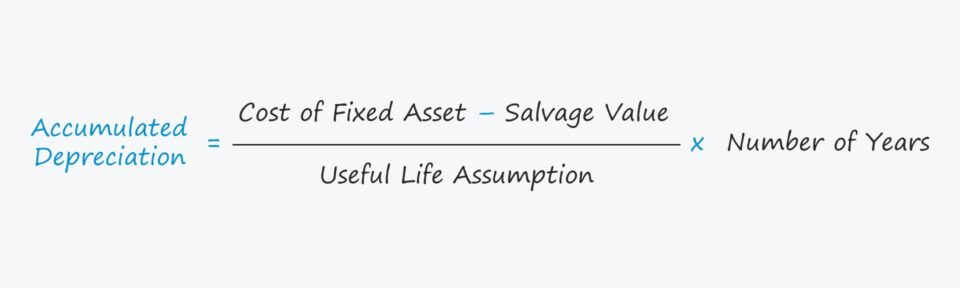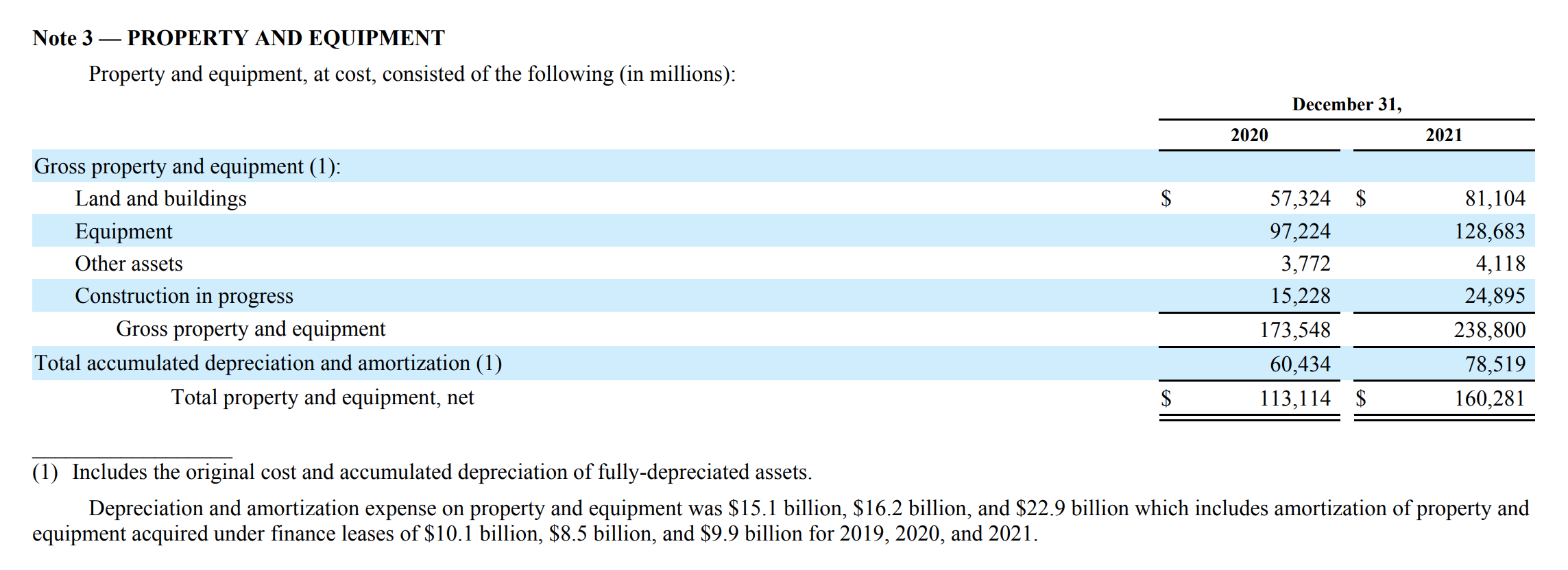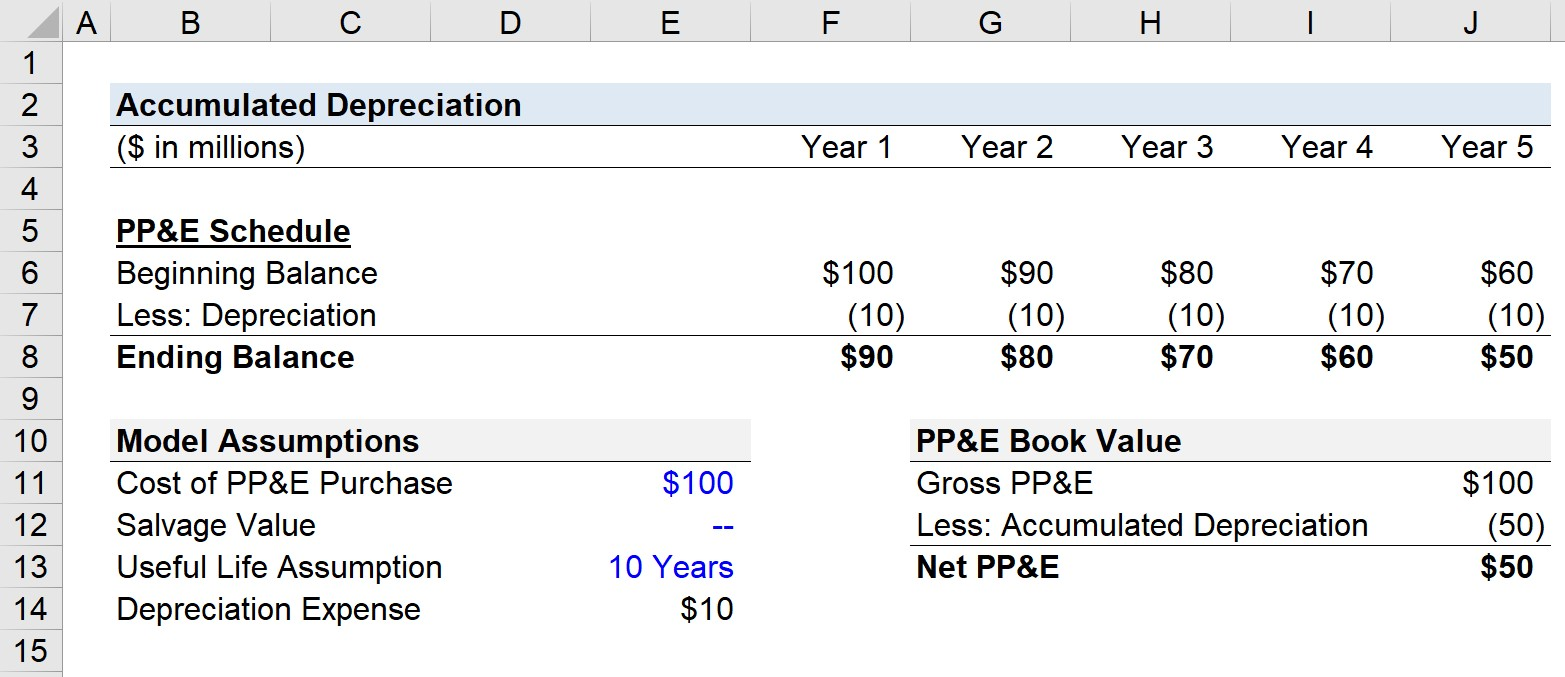Welcome to Wall Street Prep! Use code at checkout for 15% off.# Accumulated Depreciation

Guide to Understanding Accumulated Depreciation## How to Calculate Accumulated Depreciation?

In accrual accounting, the “Accumulated Depreciation” on a fixed asset refers to the sum of all depreciation expenses since the date of original purchase.

The concept of depreciation describes the allocation of the purchase of a fixed asset, or capital expenditure, over its useful life.

The purpose of depreciation is to match the timing of the purchase of a fixed asset (“cash outflow”) to the economic benefits received (“cash inflow”).

If a company decides to purchase a fixed asset (PP&E), the total cash expenditure is incurred in once instance in the current period.

Yet, the capital expenditure (Capex) must be spread across the useful life of the fixed asset per the matching principle, i.e. the number of years in which the fixed asset is expected to provide benefits.

Each period in which the depreciation expense is recorded, the carrying value of the fixed asset, i.e. the property, plant and equipment (PP&E) line item on the balance sheet, is gradually reduced.

On the income statement, the incremental depreciation expense is recognized – most often embedded within the cost of goods sold (COGS) or operating expenses line items – until reaching its salvage value, which represents the asset’s residual value at the end of the useful life assumption.

## Accumulated Depreciation Journal Entry (Debit or Credit)

While the depreciation expense is the amount recognized each period, the accumulated depreciation is the sum of all depreciation to date since purchase.

Because the accumulated depreciation account is an asset that carries a credit balance, it is considered a contra asset.

A contra asset is defined as an asset account that offsets the asset account to which it is paired, i.e. the reverse of the standard impact on the books.

Therefore, the accumulated depreciation reduces the fixed asset (PP&E) balance recorded on the balance sheet.

In most cases, fixed assets carry a debit balance on the balance sheet, yet accumulated depreciation is a contra asset account, since it offsets the value of the fixed asset (PP&E) that it is paired to.

## Accumulated Depreciation Formula

The formula for calculating the accumulated depreciation on a fixed asset (PP&E) is as follows.

Accumulated Depreciation = [(Cost of Fixed Asset Salvage Value) ÷ Useful Life Assumption] × Number of Years

Alternatively, the accumulated expense can also be calculated by taking the sum of all historical depreciation expense incurred to date, assuming the depreciation schedule is readily available.

## What is an Example of Accumulated Depreciation?

For a real world example of accumulated depreciation in the financial filings of a company, see the “Property and Equipment” section of Amazon’s 10-K report.

Starting from the gross property and equity value, the accumulated depreciation value is deducted to arrive at the net property and equipment value for the fiscal years ending 2020 and 2021.Amazon Accumulated Depreciation Example (Source: 10-K Report)

## Accumulated Depreciation Calculator

We’ll now move to a modeling exercise, which you can access by filling out the form below.Submitting...

## 1. Balance Sheet Assumptions

Suppose that a company purchased \$100 million in PP&E at the end of Year 0, which becomes the beginning balance for Year 1 in our PP&E roll-forward schedule.

The cost of the PP&E – i.e. the \$100 million capital expenditure – is not recognized all at once in the period incurred.

• Cost of PP&E Purchase = \$100 million

In order to calculate the depreciation expense, which will reduce the PP&E’s carrying value each year, the useful life and salvage value assumptions are necessary.

• Useful Life = 10 Years
• Salvage Value = \$0

## 2. Annual Depreciation Expense Calculation Example

Since the salvage value is assumed to be zero, the depreciation expense is evenly split across the ten-year useful life (i.e. “spread” across the useful life assumption).

The depreciation incurred per year comes out to \$10 million.

• Depreciation Expense = (\$100 million – \$0 million) ÷ 10 Years = \$10 million

## 3. Accumulated Depreciation Calculation Example

In our PP&E roll-forward, the depreciation expense of \$10 million is recognized across the entire forecast, which is five years in our illustrative model, i.e. half of the ten-year useful life.

By the end of Year 5, we see that the ending PP&E balance is \$50 million.

The purchased PP&E’s value declined by a total of \$50 million across the five-year time frame, which represents the accumulated depreciation on the fixed asset.

On the balance sheet, the carrying value of the net PP&E equals the gross PP&E value minus accumulated depreciation – the sum of all depreciation expenses since the purchase date – which is \$50 million.

• Accumulated Depreciation = \$50 millionStep-by-Step Online Course

### Everything You Need To Master Financial Modeling

Enroll in The Premium Package: Learn Financial Statement Modeling, DCF, M&A, LBO and Comps. The same training program used at top investment banks.